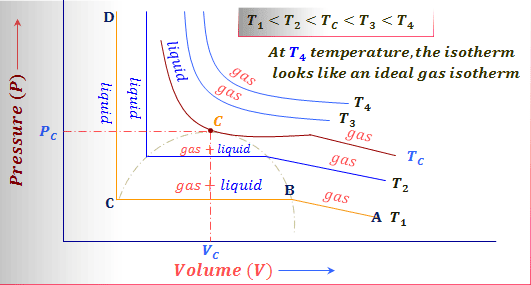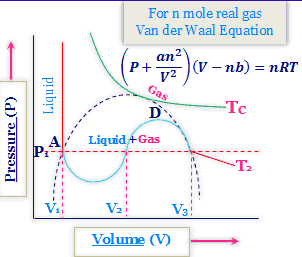# Critical constants of gas molecules

### Definitions of critical constants of gas molecules

A gas can be liquefied by lowering temperature and increasing pressure. But the influence of temperature is more important.

Most of the real gases are liquefied at ordinary pressure by the suitable lowering of the temperature. But gas cannot be liquefied unless its temperature is below a certain value depending upon the nature of the gas whatever high the pressure may be applied.

This temperature of the gas is called its critical temperature (Tc). Gas can only be liquefied when the temperature is kept below Tc of the gas molecules.

#### The critical temperature, pressure, and volume of a gas

1. Critical temperature (Tc) is the maximum temperature at which a gas can be liquefied, which is the temperature above which a liquid cannot exist.
2. Critical pressure (Pc) is the maximum pressure required to cause liquefaction at the temperature (Tc).
3. Critical volume (Vc) is the volume occupied by one mole of a gas at critical temperate (Tc) and critical pressure (Pc).

### Andrews isotherms for carbon dioxide gas

In 1869, Thomas Andrews carried out an experiment in which P - V relations of carbon dioxide gas were measured at different temperatures.Critical constant of gas
1. High temperatures, such as T4, the isotherms look like those of an Ideal gas.
2. Low temperatures, the curves have altogether different appearances. Consider, for example, a typical curve. As the pressures increase, the volume of the gas decreases in curve A to B.
3. At the point, B liquefaction commences and the volume decreases rapidly as the gas converted to a liquid with much higher density. This conversion takes place at constant pressure.
4. Point C, liquefaction is complete and thus the CD is evidence of the fact that the liquid cannot be easily compressed. Thus, we note that AB represents the gaseous state, BC, liquid, and vapor in equilibrium, and CD shows the liquid state only.
5. Still higher temperatures we get a similar type of curve as discussed in the above point, except that the horizontal portion is reduced. The pressure corresponding to this portion is higher than at lower temperatures.
6. At temperatures, Tc the horizontal portion is reduced to a mere point. At temperatures higher then Tc there is no indication of qualification at all.
For every gas can have a limit of temperature above which the gas can not be liquefied, no matter what the pressure is.

#### Continuity of state for real gas molecules

It appears from the Amagat curve at T there is discontinuity or break during the transformation of gas to liquid. But it is not so. The continuity of the states from the gas to liquid can be explained from the following Andrews isotherm ABCD at T₁.

The gas at A is heated to B at constant volume along AB. Then the gas is gradually cooled at constant P along BC, the volume is reduced considerably. The gas is again cooled at constant volume until point D is reached. Nowhere in the process liquid would appear.

At D, the system is a highly compressed gas. But from the curve, this point is the representation of the liquid state. Hence there is hardly a distinction between the liquid state and the gaseous state.

There is no line of separation between the two phases. This is known as the principle of continuity of the state.

### Pc, Vc, and Tc from Van der Walls curve

Van der Waals equation for 1-mole real gas
(P + a/V2)(V - b) = RT
or, V3 - (b + RT/P)V2 + (a/P)V - ab/P = 0

This equation has three roots in volume for given values of a, b, P and T. It found that either all the three roots are real or one is real and the other two are imaginary.

Study for schools and college coursesCritical phenomena
1. At higher temperatures and higher volume regions, the isotherms look much like the isotherms from Ideal gas law.
2. At the temperature lower than Tc the isotherm exhibits a maximum and a minimum. For certain values of pressure, the equation gives three roots of volume as V1, V2, and V3 at pressure.
The section AB and ED of the Van der Waals curve at T1 can be realized experimentally. ED represents supersaturated or supercooled vapor and AB represents superheated liquid. Both of these states are meta-stable.
These states are unstable in the seance that slight disturbances are sufficient to cause the system to revert spontaneously into the stable state with the two phases present in equilibrium.
3. The section BCD of the Van der Waals isotherms cannot be realized experimentally. In this region the slope of the P - V curve is positive. Increasing or decreasing the volume of such a system would increase or decrease in pressure. The line BCD represents the metastable state.

### Critical constants from Van der Waals equation

Again with the increase of temperature the minimum and maximum points come close to each other and at the critical point (C) both maximum and minimum coalesce. The slope and curvature both become zero at this point.
Mathematical condition of the critical point
(dP/dV)T = 0
(d²P/dV²)T = 0.

Van der Waals equation for 1 mole gas
(P + a/V²)(V - b) = RT or, P = {RT/(V - b)} - a/V².
Differentiating with respect to the volume at constant temperature gives the slope of the curve.

∴ Slope = (dP/dV)T = - {RT/(V - b)²} + 2a/V³.
Curvature = (d²P/dV²)T = {2RT/(V - b)³} - 6a/V⁴.

#### Vc from Van der Waals equation

When P = Pc, V = Vc, and T = Tc.
- {RTc/(Vc - b)2} + 2a/Vc³ = 0
or, RTc/(Vc - b)² = 2a/Vc³.

{2RTc/(Vc - b)³} - 6a/Vc⁴ = 0
or, 2RTc/(Vc - b)3 = 6a/Vc⁴
or, (Vc - b)/2 = Vc/3
∴ Vc = 3b.

#### Tc for real gas molecules

RTc/(Vc - b)² = 2a/Vc³.
Here Vc =3b.

RTc/4b² = 2a/27b³
∴ Tc = 8a/27Rb

#### Pc from Van der Waals equation

Van der Walls equation at the critical state,
Pc = RTc/(Vc - b) - a/Vc²
Again Vc = 3b and Tc = 8a/27Rb
∴ Pc = a/27b²
Problem
Calculate Van der Waals constants for ethylene. (Tc = 280.8 K and Pc = 50 atm).

a = 0.057 lit mol⁻¹ and b = 4.47 lit² atm mol⁻²

#### Compressibility factor Z for real gas molecule

Z = PV/RT
At critical point of gases
Zc = RTc/PcVc = {(a/27b²) × 3b}/{R × (8a/27Rb)} = 3/8
= 0.375

Critical coefficient = RTc/PcVc = 8/3
= 1.66

#### a and b in terms of critical constants

a and b for real gas can be determined from critical constants of gas, Tc and Pc. Vc in the expression is avoided due to difficulty in its determination.

b = Vc/3 PcVc/RTc = 3/8
or, Vc = (3/8) × (RTc/Pc)
∴ b = (1/8)(RTc/Pc)

a = Pc × 27b² = 3 × Pc × (3b)²
= 3 PcVc²
= 3Pc × (3RTc/8Pc)²
∴ a = (27/64)(R²Tc²/Pc)
Problem
The critical constants for water are 647 K, 22.09 MPa and 0.0566 dm³ mol⁻¹. What is the value of a and b?
Solution
Tc = 647 K
Pc = 22.09 Mpa = 22.09 × 10³ kPa
Vc = 0.0566 dm³ mol⁻¹
b = Vc/3 = (0.0566 dm³ mol⁻¹)/3

∴ b = 0.0189 dm³ mol⁻¹

a = 3 Pc Vc²
= 3 (22.09 × 10³) × (0.0566)²
∴ a = 213.3 kPa mol⁻²

Problem
Argon has Tc = - 122°C, Pc = 48 atm. What is the radius of the argon atom?